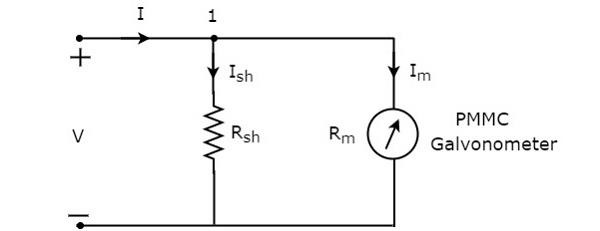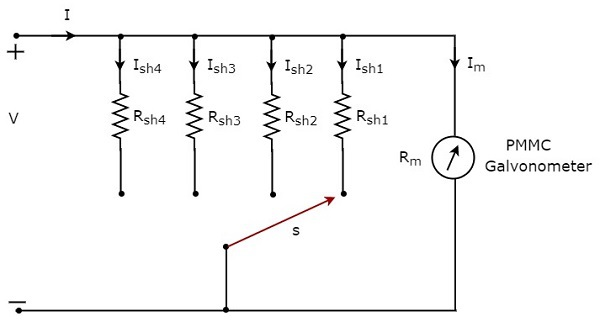# DC Ammeters

Current is the rate of flow of electric charge. If this electric charge flows only in one direction, then the resultant current is called Direct Current (DC). The instrument, which is used to measure the Direct Current called DC ammeter.

If we place a resistor in parallel with the Permanent Magnet Moving Coil (PMMC) galvanometer, then the entire combination acts as DC ammeter. The parallel resistance, which is used in DC ammeter is also called shunt resistance or simply, shunt. The value of this resistance should be considered small in order to measure the DC current of large value.

The circuit diagram of DC ammeter is shown in below figure.We have to place this DC ammeter in series with the branch of an electric circuit, where the DC current is to be measured. The voltage across the elements, which are connected in parallel is same. So, the voltage across shunt resistor, $R_{sh}$ and the voltage across galvanometer resistance, $R_{m}$ is same, since those two elements are connected in parallel in above circuit. Mathematically, it can be written as

$$I_{sh}R_{sh}=I_{m}R_{m}$$

$\Rightarrow R_{sh}=\frac{I_{m}R_{m}}{I_{sh}}$ (Equation 1)

The KCL equation at node 1 is

$$-I+I_{sh}+I_{m}=0$$

$$\Rightarrow I_{sh}=I-I_{m}$$

Substitute the value of $I_{sh}$ in Equation 1.

$R_{sh}=\frac{I_{m}R_{m}}{I-I_{m}}$(Equation 2)

Take, $I_{m}$ as common in the denominator term, which is present in the right hand side of Equation 2

$$R_{sh}=\frac{I_{m}R_{m}}{I_{m}(\frac{1}{I_{m}}-1)}$$

$\Rightarrow R_{sh}=\frac{R_{m}}{\frac{I}{I_{m}}-1}$(Equation 3)

Where,

$R_{sh}$ is the shunt resistance

$R_{m}$ is the internal resistance of galvanometer

$I$ is the total Direct Current that is to be measured

$I_{m}$ is the full scale deflection current

The ratio of total Direct Current that is to be measured, $I$ and the full scale deflection current of the galvanometer, $I_{m}$ is known as multiplying factor, m. Mathematically, it can be represented as

$m=\frac{I}{I_{m}}$(Equation 4)

$R_{sh}=\frac{R_{m}}{m-1}$(Equation 5)

We can find the value of shunt resistance by using either Equation 2 or Equation 5 based on the available data.

## Multi Range DC Ammeter

In previous section, we discussed about DC ammeter which is obtained by placing a resistor in parallel with the PMMC galvanometer. This DC ammeter can be used to measure a particular range of Direct Currents.

If we want to use the DC ammeter for measuring the Direct Currents of multiple ranges, then we have to use multiple parallel resistors instead of single resistor and this entire combination of resistors is in parallel to the PMMC galvanometer. The circuit diagram of multi range DC ammeter is shown in below figure.Place this multi range DC ammeter in series with the branch of an electric circuit, where the Direct Current of required range is to be measured. The desired range of currents is chosen by connecting the switch, s to the respective shunt resistor.

Let, $m_{1}, m_{2}, m_{3}$ and $m_{4}$ are the multiplying factors of DC ammeter when we consider the total Direct Currents to be measured as, $I_{1}, I_{2}, I_{3}$ and $I_{4}$ respectively. Following are the formulae corresponding to each multiplying factor.

$$m_{1}=\frac{I_{1}}{I_{m}}$$

$$m_{2}=\frac{I_{2}}{I_{m}}$$

$$m_{3}=\frac{I_{3}}{I_{m}}$$

$$m_{4}=\frac{I_{4}}{I_{m}}$$

In above circuit, there are four shunt resistors, $R_{sh1}, R_{sh2}, R_{sh2}$ and $R_{sh4}$. Following are the formulae corresponding to these four resistors.

$$R_{sh1}=\frac{R_{m}}{m_{1}-1}$$

$$R_{sh2}=\frac{R_{m}}{m_{2}-1}$$

$$R_{sh3}=\frac{R_{m}}{m_{3}-1}$$

$$R_{sh4}=\frac{R_{m}}{m_{4}-1}$$

The above formulae will help us find the resistance values of each shunt resistor.